GeeksforGeeks App
Open AppBrowser
Continue

# Implement dynamic queue using templates class and a circular array

In this article, we will discuss how to create a dynamic circular queue using a circular array having the following functionality:

• Front(): Get the front item from the queue.
• Back(): Get the last item from the queue.
• Push(X): Push the X in the queue at the end of the queue.
• Pop(): Delete an element from the queue.

Below is the step-by-step illustration:

• Initially, the queue is empty.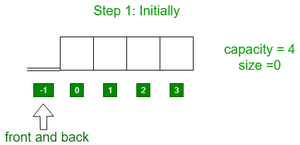• Insert element 1 to the back of the queue.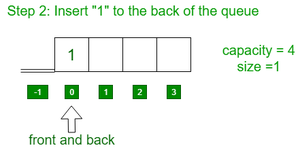• Insert elements 2, 3, 4 to the back of the queue.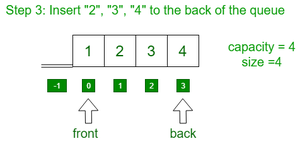• Insert elements 5 to the back of the queue.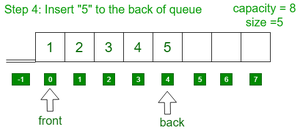• Pop 4 elements from the queue.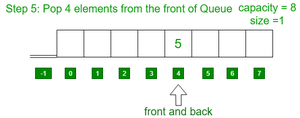• Pop 1 element from the queue.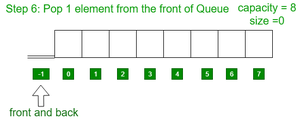Approach: The idea is to double the size of the array used every time the capacity of the array gets full and copy the elements of the previous array into the new array. Follow the steps below to solve the problem:

• Initialize 4 variables say frontIndex, backIndex, sizeVar, and capacity and an array say arr[] to implement the queue,
• Define a function say Capacity() to find the size of the current array used:
• Return the capacity.
• Define a function say size() to find the count of elements in the queue:
• Return the variable sizeVar.
• Define a function say full() to find if the queue is full or not:
• Return true if sizeVar is equal to capacity. Otherwise, return false.
• Define a function say empty() to find if the queue is empty or not:
• If frontIndex and backIndex are equal to -1 then return true. Otherwise, return false.
• Define a function say Front() to print the front element of the queue:
• Define a function say Back() to print the last element of the queue:
• Print the element of arr[BackIndex] if queue is not empty().
• Define a function say Push(X) to insert an element at the end of the queue:
• If the queue is full then double the size of the current array and copy the elements of the previous array into the new array.
• If queue is empty() then assign frontIndex = backIndex = 0 and then assign X to both arr[frontIndex] and arr[backIndex] and then increment sizeVar by one.
• Else, update backIndex as backIndex = (backIndex+1)%capacity and then assign X to arr[backIndex] and increment sizeVar by one.
• Define a function say Pop() to delete an element at the front of the queue:
• If the queue is empty print “Underflow”.
• Else if sizeVar is equal to 1 then assign -1 to frontIndex and backIndex both and then decrement sizeVar by one.
• Else, Update frontIndex as frontIndex = (frontIndex+1)%capacity and decrement sizeVar by one.

Below is the implementation of the above approach:

## C++

 `// C++ program for the above approach``#include ``using` `namespace` `std;` `// Class definition for queue``template` `<``class` `X>``class` `Queue {` `private``:``    ``// Stores the frontIndex``    ``int` `frontIndex;` `    ``// Stores the back Index``    ``int` `backIndex;` `    ``// Stores the array``    ``X* arr;` `    ``// Stores the sizeof queue``    ``int` `sizeVar;` `    ``// Stores the size of array``    ``int` `capacityVar = 4;` `public``:``    ``// Queue class constructor``    ``Queue()``    ``{``        ``arr = ``new` `X[capacityVar];``        ``frontIndex = backIndex = -1;``        ``sizeVar = 0;``    ``}` `    ``// Function Methods``    ``bool` `empty();``    ``bool` `full();``    ``void` `push(X x);``    ``void` `pop();``    ``X front();``    ``X back();``    ``int` `capacity();``    ``int` `size();``};` `// Find the capacity of queue``template` `<``class` `X>``int` `Queue::capacity()``{``    ``return` `capacityVar;``}` `// Find the number of elements``// present in Queue``template` `<``class` `X>``int` `Queue::size()``{``    ``return` `sizeVar;``}` `// Function to check if``// Queue is empty or not``template` `<``class` `X>``bool` `Queue::empty()``{``    ``if` `(frontIndex == -1``        ``&& backIndex == -1)``        ``return` `true``;``    ``else``        ``return` `false``;``}` `// Function to check if the queue``// is full or not``template` `<``class` `X>``bool` `Queue::full()``{``    ``if` `(sizeVar == capacityVar)``        ``return` `true``;``    ``else``        ``return` `false``;``}` `// Function to find the front element``// of the queue``template` `<``class` `X>``X Queue::front()``{``    ``// If queue is empty``    ``if` `(empty()) {``        ``cout << ``"Queue underflow"``             ``<< endl;``        ``abort``();``    ``}` `    ``return` `arr[frontIndex];``}` `// Function to find the last element``// of the Queue``template` `<``class` `X>``X Queue::back()``{``    ``if` `(empty()) {``        ``cout << ``"Queue underflow"``             ``<< endl;``        ``abort``();``    ``}``    ``return` `arr[backIndex];``}` `// Function to insert the element``// to the rear end of the queue``template` `<``class` `X>``void` `Queue::push(X x)``{``    ``if` `(full()) {` `        ``// If the queue is full, then``        ``// double the capacity``        ``capacityVar = capacityVar * 2;` `        ``// Initialize new array of``        ``// double size``        ``X* temp = ``new` `X[capacityVar];` `        ``// Copy the elements of the``        ``// previous array in the order of the queue``        ``for` `(``int` `i = 0, j= frontIndex; i < sizeVar; i++){``          ``temp[i] = arr[j];``          ``j = (j+1) % sizeVar;``        ``}``        ``// update the front and rear indices in the new array``          ``frontIndex = 0;``          ``backIndex =  sizeVar -1;` `        ``// Deallocate the memory``        ``// of previous array``        ``delete``[] arr;``        ``arr = temp;``    ``}` `    ``// If size is zero``    ``if` `(empty()) {` `        ``frontIndex = backIndex = 0;``        ``arr[backIndex] = x;``        ``sizeVar++;``        ``return``;``    ``}` `    ``// Increment the backIndex``    ``backIndex = (backIndex + 1) % capacityVar;``    ``arr[backIndex] = x;``    ``sizeVar++;` `    ``return``;``}` `// Function to pop an element from``// front end of the queue``template` `<``class` `X>``void` `Queue::pop()``{``    ``// If queue is empty``    ``if` `(empty()) {``        ``cout << ``"Queue underflow"``             ``<< endl;``        ``abort``();``    ``}` `    ``// If there is only one character``    ``if` `(frontIndex == backIndex) {` `        ``// Mark Queue as empty``        ``// and decrement sizeVar``        ``frontIndex = backIndex = -1;``        ``sizeVar--;``        ``return``;``    ``}` `    ``// Increment frontIndex cyclically``    ``// using modulo arithmetic``    ``frontIndex = (frontIndex + 1) % capacityVar;``    ``sizeVar--;` `    ``return``;``}` `// Driver Code``int` `main()``{``    ``// Queue initialization``    ``Queue<``int``> q;` `    ``// Iterate the range [1, 100]``    ``for` `(``int` `i = 1; i < 5; i++)``        ``q.push(i);` `    ``// Print the current capacity``    ``cout << ``"Current capacity "``        ``<< q.capacity() << endl;` `    ``// Print current size``    ``cout << ``"Current size "``        ``<< q.size() << endl;` `    ``// Print front elements of queue``    ``cout << ``"Front element "``        ``<< q.front() << endl;` `    ``// Print last element of the queue``    ``cout << ``"Rear element "``        ``<< q.back() << endl;` `    ``cout << endl;` `    ``cout << ``"Pop an element"` `<< endl;` `    ``// Pop an element from the queue``    ``q.pop();` `    ``cout << ``"Pop an element"` `<< endl;` `    ``// Pop an element from the queue``    ``q.pop();` `    ``cout << endl;` `    ``// Print the current capacity``    ``cout << ``"Current capacity "``        ``<< q.capacity() << endl;` `    ``// Print current size``    ``cout << ``"Current size "``        ``<< q.size() << endl;` `    ``// Print front elements of queue``    ``cout << ``"Front element "``        ``<< q.front() << endl;` `    ``// Print last element of the queue``    ``cout << ``"Rear element "``        ``<< q.back() << endl;``        ` `        ``cout << endl;``    ``cout << ``"Push element 5"` `<< endl;``    ``cout << ``"Push element 6"` `<< endl;``    ` `    ` `    ``q.push(5);``    ``q.push(6);``    ``cout << endl;` `    ``// Print the current capacity``    ``cout << ``"Current capacity "``        ``<< q.capacity() << endl;` `    ``// Print current size``    ``cout << ``"Current size "``        ``<< q.size() << endl;` `    ``// Print front elements of queue``    ``cout << ``"Front element "``        ``<< q.front() << endl;` `    ``// Print last element of the queue``    ``cout << ``"Rear element "``        ``<< q.back() << endl;` `    ``cout << endl;``    ``cout << ``"Push element 7"` `<< endl;``    ``q.push(7);``    ` `    ``cout << endl;` `    ``// Print the current capacity``    ``cout << ``"Current capacity "``        ``<< q.capacity() << endl;` `    ``// Print current size``    ``cout << ``"Current size "``        ``<< q.size() << endl;` `    ``// Print front elements of queue``    ``cout << ``"Front element "``        ``<< q.front() << endl;` `    ``// Print last element of the queue``    ``cout << ``"Rear element "``        ``<< q.back() << endl;` `    ``return` `0;``}`

Output:

```Current capacity 128
Current size 99
Front element 1
Rear element 99

Pop an element
Pop an element

Current capacity 128
Current size 97
Front element 3
Rear element 99```

Time Complexity: O(N)
Auxiliary Space: O(N)

My Personal Notes arrow_drop_up